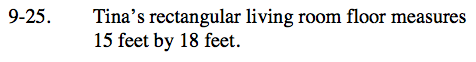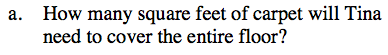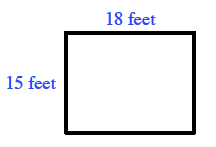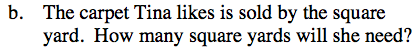### Home > CC3 > Chapter 9 > Lesson 9.1.2 > Problem9-25

9-25.
1. Tina’s rectangular living room floor measures 15 feet by 18 feet. Homework Help ✎

1. How many square feet of carpet will Tina need to cover the entire floor?

2. The carpet Tina likes is sold by the square yard. How many square yards will she need?Draw a diagram of the room.

Square feet refers to the area of the room.
Using the diagram, find the area of the room.Each square yard is equivalent to nine square feet.
Convert your square feet answer from part (a) into square yards.

Divide the total square feet by 9.

30 square yards